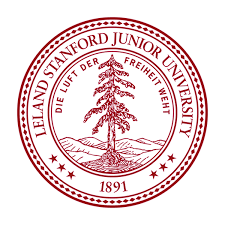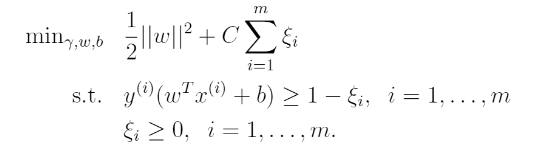# [Stanford CS229] Lecture8 - Data Splits, Models & Cross-Validation

jhbale11·2021년 7월 5일
0

## Stanford CS229 Lecture Note

목록 보기
8/8## 1. Bias/Variance

The generalization error of a hypothesis is its expected error on examples not necessarily in the training set.

Informally we define the bias of a model to be the expected generalization error even if we were to fit it to a very large training set. Thus linear model from large bias may underfit.

Apart from bias, there's a second component to the generalization error, consisting of the variance of a model fitting procedure.

Bias in Machine Learing : Algorithm has very strong preconceptions that the data could be fit by linear functions.

High Variance in Machine Learning : there's a lot of variability in the predictions this algorithm will make

Bias와 Variance를 통해서 Learning Algorithm을 끊임 없이 개선하는 것이 machine learning에서 매우 중요하다.

## 2. Regularization

To make the algorithm work for non-linearly separable datasets as well as be less sensitive to outliers, we reformulate our optimization as follows:## 3. Data Split

Train/Development/Test set

Let's suppose we are given a training set S. Given what we know about empirical risk minimization, here's what might initially seem like a algorithm, resulting from using risk minimization for model selection:

(1) Train each model M on S, to get some hypothesis h.
(2) Pick the hypotheses with the smallest training error.

But this algorithm does not work because of choosing the order of a polynomial.
Here's an algorithm that works better. In hold-out cross validation.

(1) Randomly split S into S(train) and S(cv). Here S(cv) is called the hold-out cross validation set. S(train) is usually 70% of the data, and S(cv) is usually remaining 30%.
(2) Train each model M on S(train) only to get some hypothesis h.
(3) Select and output the hypothesis h that had the smallest error on the hold out cross validation set.

By training on a set of exampe S(cv) that the models were not trained on, we obtain a better estimate of each hypothesis h's true generalization error, and can then pick the one with the smallest estimated generalization error.

Usually 30% of the data is used in the hold-oout cross validatoin set and 30% is typical choice.

Also there is method called k-fold cross validation that holds out less data each time:
(1) Randomly split S into k disjoint subsets of m/k training examples each. Let's call these subsets S1...Sk.
(2) For each model M, we evaluate it as follows:
1) For j = 1,...,k Train the model M on $S_{1}\cup...\cup S_{j-1}\cup S_{j+1}\cup ... \cup S_{k}$ to get some hypothesis $h_{ij}$.
2) Test the hypothesis $h_{ij}$ on $S_{j}$ to get error of $h_{ij}$.
3) The estimated generalization error of model M is then calculated as the average of the error of $h_{ij}$.
(3) Pick the model M with the lowest estimated generalization error, and retrain the model on the entire training set S. The resulting hypothesis is then output as our final answer.

## 4. Model Selection

.
.
.
TO BE CONTINUED...